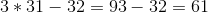Example Questions

Example Question #21 : Order Of Operations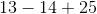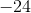Explanation:

When doing operations of subtraction and addition, there is no priority but we must work from left to right. The difference on the left is. Sinceis greater thanand is negative, we treat as a normal subtraction problem. The same applies for the right side in whichis greater thanand is positive. The answer is.

Example Question #22 : Order Of OperationsExplanation:

There is multiplication and addition present. Remember PEMDAS. Multiplication comes first followed by addition.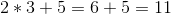Example Question #23 : Order Of Operations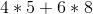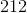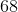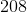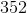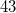Explanation:

There is multiplication and addition present. Remember PEMDAS. Multiplication comes first followed by addition. Since there are two multiplication operations, we work from left to right then finally add the products.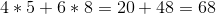Example Question #24 : Order Of Operations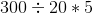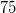Explanation:

When there's multiplication and division, they have the same priority. We will work from left to right.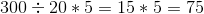Example Question #25 : Order Of Operations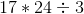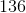Explanation:

When there's multiplication and division, they have the same priority. We will work from left to right.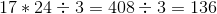Example Question #26 : Order Of Operations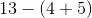Explanation:

Although there is just addition and subtraction, a paranthesis is present. According to PEMDAS, paranthesis has priority over all operations.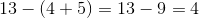Example Question #27 : Order Of Operations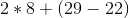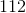Explanation:

Even there is multiplication, addition and subtraction, a paranthesis is present. According to PEMDAS, paranthesis has priority over all operations.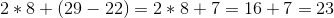Example Question #28 : Order Of Operations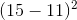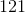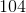Explanation:

In PEMDAS, the paranthesis comes first followed by the exponent. We have subtraction so the difference is. We square this to get.

Example Question #29 : Order Of Operations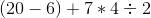Explanation:

In PEMDAS, the paranthesis comes first.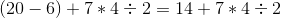Next, multiplication and division is next and we work from left to right. Lastly, we add.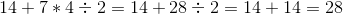Example Question #30 : Order Of Operations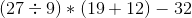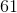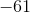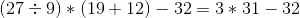Next, multiplication has priority over subtraction.